# 一、GCN简介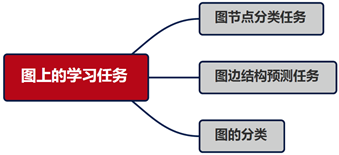GNN模型主要研究图节点的表示（Graph Embedding），图边结构预测任务和图的分类问题，后两个任务也是基于Graph Embedding展开的。目前论文重点研究网络的可扩展性、动态性、加深网络。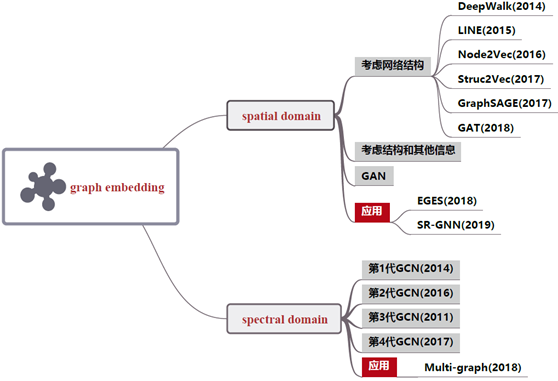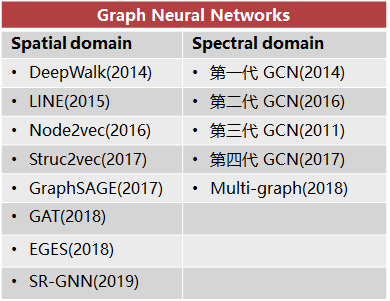• 加深网络: 研究表明随着网络层数增加，模型性能急剧下降
• 感受野:节点的感受野是指一组节点，包括中心节点和其近邻节点，有些节点可能只有一个近邻，而有些节点却有数千个近邻
• 可扩展性:大部分图神经网络并不能很好地扩展到大型图上。
• 动态性和异质性:大多数当前的图神经网络都处理静态同质图。一方面，假设图架构是固定的。另一方面，假设图的节点和边来自同一个来源。

GCN模型具备深度学习的三种性质：

• 层级结构（特征一层一层抽取，一层比一层更抽象，更高级）
• 非线性变换 （增加模型的表达能力）
• 端对端训练（不需要再去定义任何规则，只需要给图的节点一个标记，让模型自己学习，融合特征信息和结构信息。）

GCN的四个特征：

• GCN 是对卷积神经网络在 graph domain 上的自然推广
• 它能同时对节点特征信息与结构信息进行端对端学习，是目前对图数据学习任务的最佳选择。
• 图卷积适用性极广，适用于任意拓扑结构的节点与图。
• 在节点分类与边预测等任务上，在公开数据集上效果要远远优于其他方法。

# 二、spectral  domain

## 1.离散卷积是什么，在CNN中发挥什么作用？

CNN中的卷积本质上就是利用一个共享参数的过滤器（kernel），**通过计算中心像素点以及相邻像素点的加权和来构成feature map实现空间特征的提取**，当然加权系数就是卷积核的权重系数。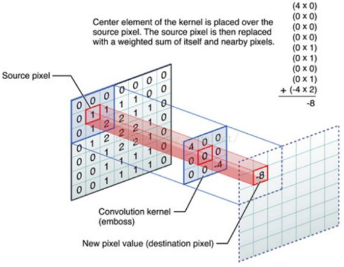## 2.GCN中的Graph是指什么？为什么要研究GCN?

CNN是Computer Vision里的法宝，效果为什么好呢？原因在上面已经分析过了，可以很有效地提取空间特征。但是有一点需要注意：**CNN处理的图像或者视频数据中像素点（pixel）是排列成成很整齐的矩阵**（如图2所示，也就是很多论文中所提到的Euclidean Structure）。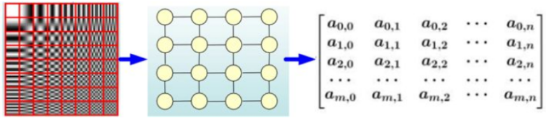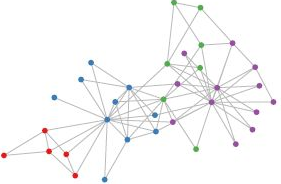（1）因为CNN无法处理非欧几里得结构的数据，学术上的表述是传统的离散卷积在非欧几里得结构的数据上无法保持平移不变性。通俗来说就是在拓扑图中每个顶点的相邻顶点数目都可能不同，那就没办法用一个同样尺寸的卷积核来进行卷积操作；

（2）广义来讲任何数据在赋范空间内都可以建立拓扑关联，谱聚类就是应用了这样的思想，所以说拓扑连接是一种广义的数据结构，GCN有很大的应用空间。

## 3.提供拓扑图空间特征的两种方式

### （1）vertex domain（spatial domain）

a.按照什么条件去找中心vertex的neighbors，如何确定receptive filed？

b.确定了receptive field之后，按照什么方式处理包含不同数目neighbors的特征？

Learning Convolutional Neural Networks for Graphs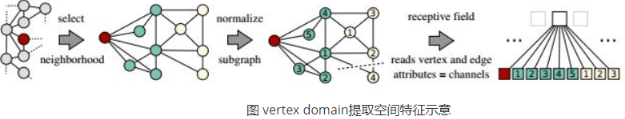c.每个顶点提取出来的neighbors不同，使得计算处理必须针对每个顶点

d.提取特征的效果可能没有卷积好

### （2）spectral domain

• Q1 什么是Spectral graph theory？
• 简单的概括就是**借助于图的拉普拉斯矩阵的特征值和特征向量来研究图的性质**
• Q2 GCN为什么要利用Spectral graph theory？
• 这应该是看论文过程中读不懂的核心问题了，要理解这个问题需要大量的数学定义及推导，短期内难以驾驭，有时间再补

## 4.什么是拉普拉斯矩阵？为什么GCN要用拉普拉斯矩阵？

Graph Fourier Transformation及Graph Convolution定义都用到图的拉普拉斯矩阵，那么首先来介绍一下拉普拉斯矩阵。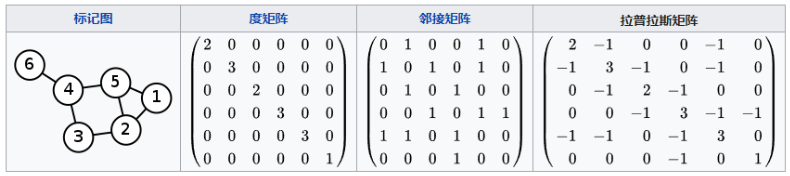NO.1：Combinatorial Laplacian：$L=D-A$

NO.2：Symmetric normalized Laplacian：$L^{sys}=D^{-1/2}LD^{-1/2}$

NO.3：Random walk normalized Laplacian：$L^{rw}=D^{-1}L$

(1)拉普拉斯矩阵是对称矩阵，可以进行特征分解（谱分解），这就和GCN的spectral domain对应上了

(2)拉普拉斯矩阵只在中心顶点和一阶相连的顶点上（1-hop neighbor）有非0元素，其余之处均为0

(3)通过拉普拉斯算子与拉普拉斯矩阵进类比（详见第6节）

## 5.拉普拉斯矩阵的谱分解（特征分解）

• 对称矩阵一定n个线性无关的特征向量
• 半正定矩阵的特征值一定非负
• 对阵矩阵的特征向量相互正交，即所有特征向量构成的矩阵为正交矩阵。

$$L=U\begin{pmatrix} \lambda_1& & \\ & ...& \\ & & \lambda_n \end{pmatrix}U^{-1}$$

$$L=U\begin{pmatrix} \lambda_1& & \\ & ...& \\ & & \lambda_n \end{pmatrix}U^{T}$$

## 6.如何从传统的傅里叶变换、卷积类比到Graph上的傅里叶变换及卷积？

### （1）推广傅里叶变换

• (a)Graph上的傅里叶变换

$$F(W)=F[(t)]=\int f(t)e^{-iwt}dt$$

$e^{-iwt}$是拉普拉斯算子的特征函数，$w$就和特征值有关。

$LV=\lambda V$ $L$是拉普拉斯矩阵，$V$是其特征向量

$$F(\lambda _l)=\hat f(\lambda _l)=\sum _{i=1}^Nf(i)u_l^{*}(i)$$

$\lambda_l$是拉普拉斯矩阵的第$l$个特征向量

$f$是Graph上的$N$维向量，$f(i)$与Graph的顶点一一对应，$u_l(i)$表示第$l$个特征向量的第$i$个分量，那么特征值（频率）$\lambda _l$下的，$f$的Graph傅里叶变换就是与$\lambda_l$对应的特征向量$u_l$进行内积运算。

**利用矩阵乘法将Graph上的傅里叶变换推广到矩阵形式：**

$$\begin{pmatrix} \hat f(\lambda_1) \\ \hat f(\lambda_2) \\ ... \\ \hat f(\lambda_N) \end{pmatrix}=\begin{pmatrix} u_1(1)& u_1(2)& u_1(N)\\ u_2(1)& u_2(2)& u_2(N)\\...& ...& ...\\ u_N(1)& u_N(2)& u_N(N)\\ \end{pmatrix}\begin{pmatrix} f(1)\\ f(2) \\ ...\\ f(N) \\ \end{pmatrix}$$

• (b)Graph上的傅里叶逆变换

$$F^{-1}(F(w))=\frac{1}{2 \pi}\int F(w)e^{iwt}dw$$

$$f(i)=\sum _{i=1}^N\hat f(\lambda_i)u_l(i)$$

**利用矩阵乘法将Graph上的傅里叶逆变换推广到矩阵形式：**

$$\begin{pmatrix} f(1) \\ f(2) \\ ... \\ f(N) \end{pmatrix}=\begin{pmatrix} u_1(1)& u_2(1)& u_N(1)\\ u_1(2)& u_2(2)& u_N(2)\\...& ...& ...\\ u_1(N)& u_2(N)& u_N(N)\\ \end{pmatrix}\begin{pmatrix} \hat f(\lambda_1)\\ \hat f(\lambda_2) \\ ...\\ \hat f(\lambda_N) \\ \end{pmatrix}$$

### （2）推广卷积

$f*h=F^{-1}[\hat f(w) \hat h(w)]=\frac {1}{2\pi} \int \hat f(w) \hat h(w) e^{iwt}dw$

$f$的傅里叶变换为$\hat f=U^Tf$

$$\begin{pmatrix} \hat h( \lambda_1)& & \\ & ...& \\ & & \hat h( \lambda_n) \end{pmatrix}$$

$\hat h(\lambda_l)=\sum ^N _{i=1}h(i)u_l^*(i)$是根据需要设计的卷积核$h$在Graph上的傅里叶变换

## 7.为什么拉普拉斯矩阵的特征向量可以作为傅里叶变换的基？特征值表示频率？

### （1）为什么拉普拉斯矩阵的特征向量可以作为傅里叶变换的基？

graph傅里叶变换也把graph上定义的任意向量 ![[公式]](https://www.zhihu.com/equation?tex=f) ，表示成了拉普拉斯矩阵特征向量的线性组合，即：

$f=\hat f(\lambda_1)u_1+\hat f(\lambda_2)u_2+...+\hat f(\lambda _n)u_n$

## 8.深度学习中的图卷积

### （1）第一代GCN

$y_{output} = \sigma(Ug_{\theta}(\Lambda)U^Tx)$

(为避免混淆，下面称$g_{\theta}(\Lambda )$是卷积核，$Ug_{\theta}(\Lambda)U$的运算结果为卷积运算矩阵)

$g_{\theta}(\Lambda)=\begin{pmatrix} \theta_1& & \\ & ...& \\ & & \theta_n \end{pmatrix}$

$\Theta=(\theta_1,\theta_2,...,\theta_n$就跟三层神经网络中的weight一样是任意的参数，通过初始化赋值然后利用误差反向传播进行调整，$x$就是graph上对应于每个顶点的feature vector（由数据集提取特征构成的向量）

• 每一次前向传播，都要计算$U、g_{\theta}(\Lambda)、U^T$三者的矩阵乘积，特别是对于大规模的graph，计算的代价较高，复杂度为$O(n^2)$
• 卷积核不具有spatial localization
• 卷积核需要n个参数

### （2）第二代GCN

$g_{\theta}(\Lambda)=\left(\begin{array}{cccc}{\sum_{j=0}^{K} \alpha_{j} \lambda_{1}^{j}} & {} & {} & {} \\ {} & {\ddots} & {} & {} \\ {} & {} & {} & {\sum_{j=0}^{K} \alpha_{j} \lambda_{n}^{j}}\end{array}\right)$

$\left(\begin{array}{cccc}{\sum_{j=0}^{K} \alpha_{j} \lambda_{1}^{j}} & {} & {} \\ {} & {\ddots} & {} \\ {} & {} & {\sum_{j=0}^{K} \alpha_{j} \lambda_{n}^{j}}\end{array}\right)=\sum_{j=0}^{K} \alpha_{j} \Lambda^{j}$

$U \sum_{j=0}^{K} \alpha_{j} \Lambda^{j} U^{T}=\sum_{j=0}^{K} \alpha_{j} U \Lambda^{j} U^{T}=\sum_{j=0}^{K} \alpha_{j} L^{j}$

$y_{output}$就变成了$y_{\text {output}}=\sigma\left(\sum_{j=0}^{K} \alpha_{j} L^{j} x\right)$

• a.卷积核只有K个参数，一般K远小于n，参数的复杂度被大大降低了；
• b.矩阵变换后，不需要做特征分解了，直接用拉普拉斯矩阵L进行变换，然而由于要计算$L^j$，计算复杂度还是$O(n^2)$
• c.卷积核具有很好的spatial localization，特别的，K就是卷积核的receptive field，也就是说每次卷积会将中心节点K-hop neighbor上的feature进行加权求和，权系数就是$\alpha_k$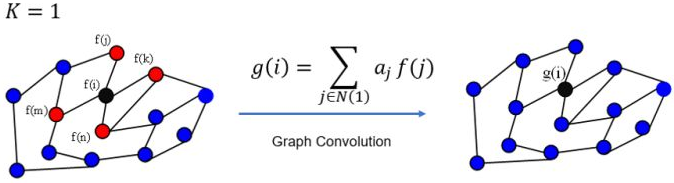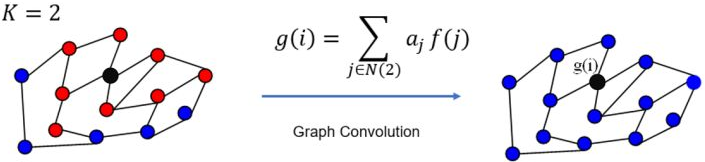• 参数太少了，只有$K$个，使得模型无法很好地实现在同阶的邻域上分配不同的权重给不同的邻居（也就是GAT论文里说的 enable specifying different weights to different nodes in a neighborhood）

### （3）第三代GCN---降低计算复杂度

$g_{\theta}(\Lambda)=\sum_{k=0}^{K-1} \beta_{k} T_{k}(\tilde{\Lambda})$

$\beta_k$是Chebyshev多项式的系数

$T_{k}(\tilde{\Lambda})$是取$\tilde{\Lambda}=2 \Lambda / \lambda_{\max }-I$的Chebyshev多项式，进行这个shift变换的原因是Chebyshev多项式的输入要在[-1,1]之间。由Chebyshev多项式的性质，可以得到如下的递推公式：

$T_{k}(\tilde{\Lambda}) x=2 \tilde{\Lambda} T_{k-1}(\tilde{\Lambda}) x-T_{k-2}(\tilde{\Lambda}) x$

$T_{0}(\tilde{\Lambda})=I, T_{1}(\tilde{\Lambda})=\tilde{\Lambda}$

[$T_0(x)=1,T_1(x)=x,T_k(x)=2xT_{k-1}(x)-T_{k-2}(x)$]

### （4）第四代GCN

$$y_{out} = \sigma(Ug_\theta(\Lambda)U^\top x) \\ Ug_\theta(\Lambda)U^\top x \approx \sum^1_{k=0}\theta_kT_k(\tilde{\Lambda})x =\theta_{0} x+\theta_1\left(L-I\right) x=\theta_0 x-\theta_1 D^{-\frac{1}{2}} A D^{-\frac{1}{2}} x$$

$$Ug_\theta(\Lambda)U^\top x \approx \theta\left(I+D^{-\frac{1}{2}} A D^{-\frac{1}{2}}\right) x$$

$$Y=\sigma\left(\tilde{D}^{-\frac{1}{2}} \tilde{A} \tilde{D}^{-\frac{1}{2}} X \Theta\right)$$

## 9.关于有向图问题

（1）要想保持理论上的完美，就需要重新定义图的邻接关系，保持对称性

（2）如果只是为了应用，有其他形式的GCN或者GAT可以处理有向图

# 三、经典算法

（一）Spectral domain

## 1.半监督学习节点分类---两层GCN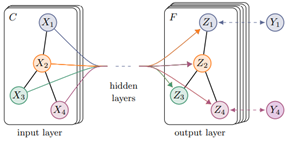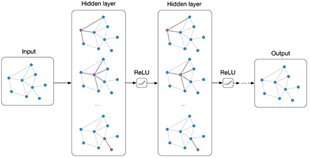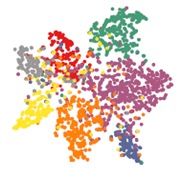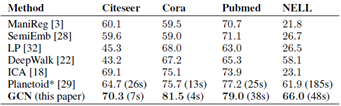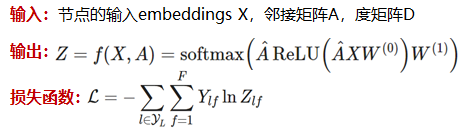## 2.基于多图卷积网络的自行车流量预测

• 构建和融合多图：定义站点间不同关系并融合得到Graph。
• GCN+encoder-decoder模型：生成包含时间、空间、流量等信息的embeddingMLP：
• 结合embedding和节假日、天气等其他特征去预测站点流量。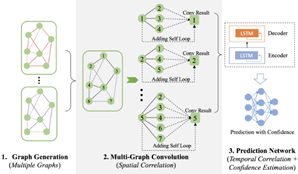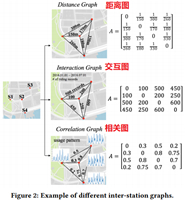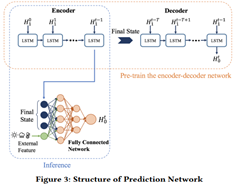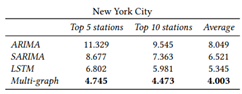（二）Spatial domain

## 1.DeepWalk( Random walk + Word2vec)

• Transductive Learning
• Corpus : Graph作为”语料库”;随机游走(采样)构建”句子”
• 可并行进行多个随机游走
• 应用：阿里提出了EGES(在DeepWalk的基础上引入物品信息)，用于生成物品的embeddings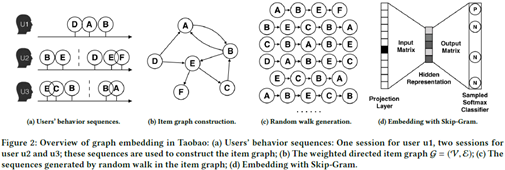DeepWalk的主要问题是它缺乏泛化能力。 每当有新节点加入到图中时，它必须重新训练模型以正确表示该节点（ 直推式学习 ）。 因此，这种GNN不适用于图中节点不断变化的动态图。

## 2.GraphSAGE( Graph SAmple and aggreGatE)

• Inductive Learning
• Deepwalk,LINE, node2vec, SDNE等模型能够高效地得到每个节点的embedding。然而，这些方法无法有效适应动态图中新增节点的特性， 往往需要从头训练或至少局部重训练。
• 通过对节点的本地邻域中的特征进行采样和聚合（平均/LSTM/pooling聚合）来生成嵌入，而不是为每个节点训练单个嵌入。
• 聚合器参数和权重变量的学习：有监督情况下，可以使用每个节点的预测label和真实label的交叉熵作为损失函数；无监督情况下，可以假设相邻节点的输出embedding应当尽可能相近，保证相邻节点的embedding的相似度尽量大的情况下，保证不相邻节点的embedding的期望相似度尽可能小。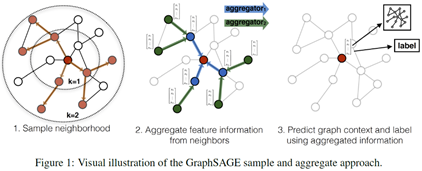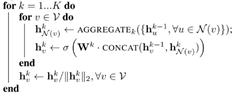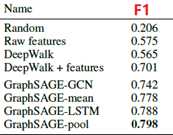## 3.GAT

• 学习注意力权重（Learn attention weights）
• 相似性注意力（Similarity-based attention）
• 注意力引导的随机游走（Attention-guided walk）

• 给定一个图中节点$v_0$ 和$v_0$的邻居节点$\left\{v_{1}, \cdots, v_{\vert\Gamma_{v_{0}}\vert}\right\} \in \Gamma_{v_{0}}$(这里的 $\Gamma_{v_{0}}$ 和GraphSAGE博文中的 $\mathcal{N}(v_0)$ 表示一个意思)。注意力机制被定义为将$\Gamma_{v_{0}}$中每个节点映射到相关性得分(relevance score)的函数$f^{\prime} :\left\{v_{0}\right\} \times \Gamma_{v_{0}} \rightarrow[0,1]$，相关性得分表示该邻居节点的相对重要性。满足：$\sum_{i=1}^{\vert\Gamma_{v_{0}}\vert} f^{\prime}\left(v_{0}, v_{i}\right)=1$

# 四、举例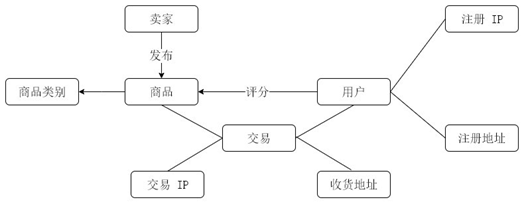• 节点分类—反欺诈：因为图中每个节点都拥有自己的特征信息。通过该特征信息，我们可以构建一个风控系统，如果交易节点所关联的用户 IP 和收货地址与用户注册 IP 和注册地址不匹配，那么系统将有可能认为该用户存在欺诈风险。
• 边结构预测—商品推荐：图中每个节点都具有结构信息。如果用户频繁购买某种类别商品或对某种类别商品评分较高，那么系统就可以认定该用户对该类商品比较感兴趣，所以就可以向该用户推荐更多该类别的商品。

【1】动手实现简单的GCN网络

【4】GNN 教程：GAT

posted @ 2019-08-05 22:56  nxf_rabbit75  阅读(13488)  评论(1编辑  收藏